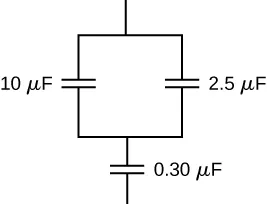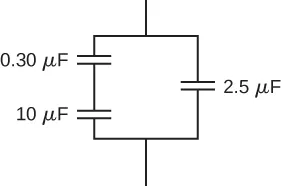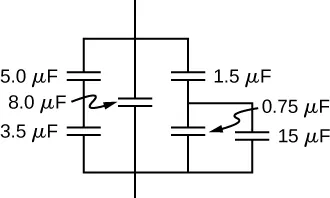University Physics Volume 2

# Problems

### 8.1Capacitors and Capacitance

19.

What charge is stored in a $180.0-μF180.0-μF$ capacitor when 120.0 V is applied to it?

20.

Find the charge stored when 5.50 V is applied to an 8.00-pF capacitor.

21.

Calculate the voltage applied to a $2.00-μF2.00-μF$ capacitor when it holds $3.10μC3.10μC$ of charge.

22.

What voltage must be applied to an 8.00-nF capacitor to store 0.160 mC of charge?

23.

What capacitance is needed to store $3.00μC3.00μC$ of charge at a voltage of 120 V?

24.

What is the capacitance of a large Van de Graaff generator’s terminal, given that it stores 8.00 mC of charge at a voltage of 12.0 MV?

25.

The plates of an empty parallel-plate capacitor of capacitance 5.0 pF are 2.0 mm apart. What is the area of each plate?

26.

A 60.0-pF vacuum capacitor has a plate area of $0.010m20.010m2$. What is the separation between its plates?

27.

A set of parallel plates has a capacitance of $5.0µF5.0µF$. How much charge must be added to the plates to increase the potential difference between them by 100 V?

28.

Consider Earth to be a spherical conductor of radius 6400 km and calculate its capacitance.

29.

If the capacitance per unit length of a cylindrical capacitor is 20 pF/m, what is the ratio of the radii of the two cylinders?

30.

An empty parallel-plate capacitor has a capacitance of $20µF20µF$. How much charge must leak off its plates before the voltage across them is reduced by 100 V?

### 8.2Capacitors in Series and in Parallel

31.

A 4.00-pF is connected in series with an 8.00-pF capacitor and a 400-V potential difference is applied across the pair. (a) What is the charge on each capacitor? (b) What is the voltage across each capacitor?

32.

Three capacitors, with capacitances of $C1=2.0μF,C1=2.0μF,$$C2=3.0μFC2=3.0μF$, and $C3=6.0μF,C3=6.0μF,$ respectively, are connected in parallel. A 500-V potential difference is applied across the combination. Determine the voltage across each capacitor and the charge on each capacitor.

33.

Find the total capacitance of this combination of series and parallel capacitors shown below.34.

Suppose you need a capacitor bank with a total capacitance of 0.750 F but you have only 1.50-mF capacitors at your disposal. What is the smallest number of capacitors you could connect together to achieve your goal, and how would you connect them?

35.

What total capacitances can you make by connecting a $5.00-μF5.00-μF$ and a $8.00-μF8.00-μF$ capacitor?

36.

Find the equivalent capacitance of the combination of series and parallel capacitors shown below.37.

Find the net capacitance of the combination of series and parallel capacitors shown below.38.

A 40-pF capacitor is charged to a potential difference of 500 V. Its terminals are then connected to those of an uncharged 10-pF capacitor. Calculate: (a) the original charge on the 40-pF capacitor; (b) the charge on each capacitor after the connection is made; and (c) the potential difference across the plates of each capacitor after the connection.

39.

A $2.0-μF2.0-μF$ capacitor and a $4.0-μF4.0-μF$ capacitor are connected in series across a 1.0-kV potential. The charged capacitors are then disconnected from the source and connected to each other with terminals of like sign together. Find the charge on each capacitor and the voltage across each capacitor.

### 8.3Energy Stored in a Capacitor

40.

How much energy is stored in an $8.00-μF8.00-μF$ capacitor whose plates are at a potential difference of 6.00 V?

41.

A capacitor has a charge of $2.5μC2.5μC$ when connected to a 6.0-V battery. How much energy is stored in this capacitor?

42.

How much energy is stored in the electrical field of a metal sphere of radius 2.0 m that is kept at a 10.0-V potential?

43.

(a) What is the energy stored in the $10.0-μF10.0-μF$ capacitor of a heart defibrillator charged to $9.00×103V9.00×103V$ ? (b) Find the amount of the stored charge.

44.

In open-heart surgery, a much smaller amount of energy will defibrillate the heart. (a) What voltage is applied to the $8.00-μF8.00-μF$ capacitor of a heart defibrillator that stores 40.0 J of energy? (b) Find the amount of the stored charge.

45.

A $165-μF165-μF$ capacitor is used in conjunction with a dc motor. How much energy is stored in it when 119 V is applied?

46.

Suppose you have a 9.00-V battery, a $2.00-μF2.00-μF$ capacitor, and a $7.40-μF7.40-μF$ capacitor. (a) Find the charge and energy stored if the capacitors are connected to the battery in series. (b) Do the same for a parallel connection.

47.

An anxious physicist worries that the two metal shelves of a wood frame bookcase might obtain a high voltage if charged by static electricity, perhaps produced by friction. (a) What is the capacitance of the empty shelves if they have area $1.00×102m21.00×102m2$ and are 0.200 m apart? (b) What is the voltage between them if opposite charges of magnitude 2.00 nC are placed on them? (c) To show that this voltage poses a small hazard, calculate the energy stored. (d) The actual shelves have an area 100 times smaller than these hypothetical shelves with a connection to the same voltage. Are his fears justified?

48.

A parallel-plate capacitor is made of two square plates 25 cm on a side and 1.0 mm apart. The capacitor is connected to a 50.0-V battery. With the battery still connected, the plates are pulled apart to a separation of 2.00 mm. What are the energies stored in the capacitor before and after the plates are pulled farther apart? Why does the energy decrease even though work is done in separating the plates?

49.

Suppose that the capacitance of a variable capacitor can be manually changed from 100 pF to 800 pF by turning a dial, connected to one set of plates by a shaft, from $0°0°$ to $180°180°$. With the dial set at $180°180°$ (corresponding to $C=800pFC=800pF$), the capacitor is connected to a 500-V source. After charging, the capacitor is disconnected from the source, and the dial is turned to $0°0°$. If friction is negligible, how much work is required to turn the dial from $180°180°$ to $0°0°$ ?

### 8.4Capacitor with a Dielectric

50.

Show that for a given dielectric material, the maximum energy a parallel-plate capacitor can store is directly proportional to the volume of dielectric.

51.

An air-filled capacitor is made from two flat parallel plates 1.0 mm apart. The inside area of each plate is $8.0cm28.0cm2$. (a) What is the capacitance of this set of plates? (b) If the region between the plates is filled with a material whose dielectric constant is 6.0, what is the new capacitance?

52.

A capacitor is made from two concentric spheres, one with radius 5.00 cm, the other with radius 8.00 cm. (a) What is the capacitance of this set of conductors? (b) If the region between the conductors is filled with a material whose dielectric constant is 6.00, what is the capacitance of the system?

53.

A parallel-plate capacitor has charge of magnitude $9.00μC9.00μC$ on each plate and capacitance $3.00μF3.00μF$ when there is air between the plates. The plates are separated by 2.00 mm. With the charge on the plates kept constant, a dielectric with $κ=5κ=5$ is inserted between the plates, completely filling the volume between the plates. (a) What is the potential difference between the plates of the capacitor, before and after the dielectric has been inserted? (b) What is the electrical field at the point midway between the plates before and after the dielectric is inserted?

54.

Some cell walls in the human body have a layer of negative charge on the inside surface. Suppose that the surface charge densities are $±0.50×10−3C/m2±0.50×10−3C/m2$, the cell wall is $5.0×10−9m5.0×10−9m$ thick, and the cell wall material has a dielectric constant of $κ=5.4κ=5.4$. (a) Find the magnitude of the electric field in the wall between two charge layers. (b) Find the potential difference between the inside and the outside of the cell. Which is at higher potential? (c) A typical cell in the human body has volume $10−16m3.10−16m3.$ Estimate the total electrical field energy stored in the wall of a cell of this size when assuming that the cell is spherical. (Hint: Calculate the volume of the cell wall.)

55.

A parallel-plate capacitor with only air between its plates is charged by connecting the capacitor to a battery. The capacitor is then disconnected from the battery, without any of the charge leaving the plates. (a) A voltmeter reads 45.0 V when placed across the capacitor. When a dielectric is inserted between the plates, completely filling the space, the voltmeter reads 11.5 V. What is the dielectric constant of the material? (b) What will the voltmeter read if the dielectric is now pulled away out so it fills only one-third of the space between the plates?

### 8.5Molecular Model of a Dielectric

56.

Two flat plates containing equal and opposite charges are separated by material 4.0 mm thick with a dielectric constant of 5.0. If the electrical field in the dielectric is 1.5 MV/m, what are (a) the charge density on the capacitor plates, and (b) the induced charge density on the surfaces of the dielectric?

57.

For a Teflon™-filled, parallel-plate capacitor, the area of the plate is $50.0cm250.0cm2$ and the spacing between the plates is 0.50 mm. If the capacitor is connected to a 200-V battery, find (a) the free charge on the capacitor plates, (b) the electrical field in the dielectric, and (c) the induced charge on the dielectric surfaces.

58.

Find the capacitance of a parallel-plate capacitor having plates with a surface area of $5.00m25.00m2$ and separated by 0.100 mm of Teflon™.

59.

(a) What is the capacitance of a parallel-plate capacitor with plates of area $1.50m21.50m2$ that are separated by 0.0200 mm of neoprene rubber? (b) What charge does it hold when 9.00 V is applied to it?

60.

Two parallel plates have equal and opposite charges. When the space between the plates is evacuated, the electrical field is $E=3.20×105V/mE=3.20×105V/m$. When the space is filled with dielectric, the electrical field is $E=2.50×105V/mE=2.50×105V/m$. (a) What is the surface charge density on each surface of the dielectric? (b) What is the dielectric constant?

61.

The dielectric to be used in a parallel-plate capacitor has a dielectric constant of 3.60 and a dielectric strength of $1.60×107V/m1.60×107V/m$. The capacitor has to have a capacitance of 1.25 nF and must be able to withstand a maximum potential difference 5.5 kV. What is the minimum area the plates of the capacitor may have?

62.

When a 360-nF air capacitor is connected to a power supply, the energy stored in the capacitor is $18.5μJ18.5μJ$. While the capacitor is connected to the power supply, a slab of dielectric is inserted that completely fills the space between the plates. This increases the stored energy by $23.2μJ23.2μJ$. (a) What is the potential difference between the capacitor plates? (b) What is the dielectric constant of the slab?

63.

A parallel-plate capacitor has square plates that are 8.00 cm on each side and 3.80 mm apart. The space between the plates is completely filled with two square slabs of dielectric, each 8.00 cm on a side and 1.90 mm thick. One slab is Pyrex glass and the other slab is polystyrene. If the potential difference between the plates is 86.0 V, find how much electrical energy can be stored in this capacitor.

Order a print copy

As an Amazon Associate we earn from qualifying purchases.